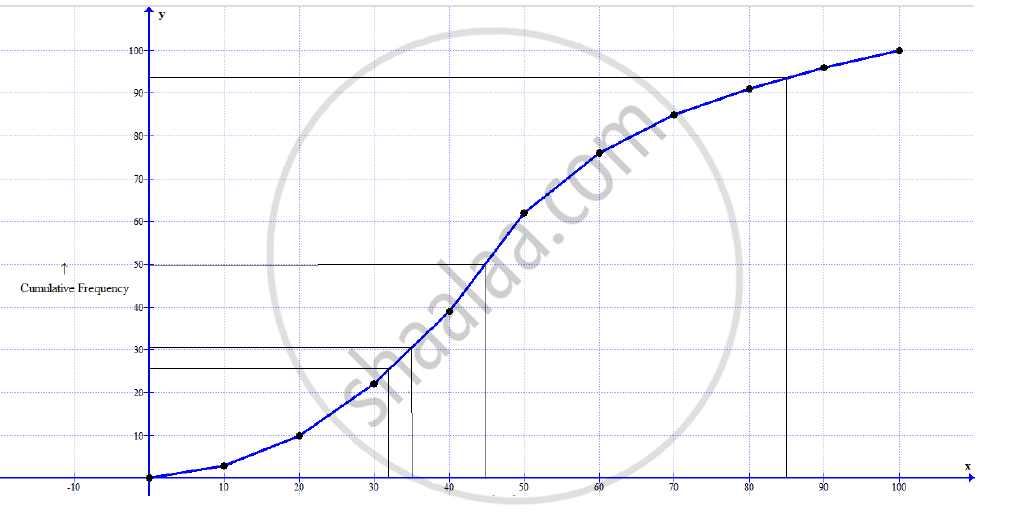Share

# The Marks Obtained by 100 Students in a Mathematics Test Are Given Below: Draw an Ogive for the Given Distribution on a Graph Sheet. - ICSE Class 10 - Mathematics

ConceptOgives (Cumulative Frequency Graphs)

#### Question

The marks obtained by 100 students in a Mathematics test are given below:

 Marks 0-10 10-20 20-30 30-40 40-50 50-60 60-70 70-80 80-90 90-100 No. ofstudents 3 7 12 17 23 14 9 6 5 4

Draw an ogive for the given distribution on a graph sheet.

Use a scale of 2 cm = 10 units on both axes.

Use the ogive to estimate the:

1) Median.

2) Lower quartile.

3) A number of students who obtained more than 85% marks in the test.

4) A number of students who did not pass in the test if the pass percentage was 35.

#### Solution

Draw the cumulative frequency table.

 Marks Number of Students (Frequency) Cumulative Frequency 0-10 3 3 10-20 7 10 20-30 12 22 30-40 17 39 40-50 23 62 50-60 14 76 60-70 9 85 70-80 6 91 80-90 5 96 90-100 4 100Scale: On x-axis, 1 unit = 10 marks, On y-axis, 1 unit = 10 students

1) Median = (N/2)^"th" term = (100/2)^"th" term = 50^"th term"

Draw a horizontal line through mark 50 on the y-axis. The, draw a vertical line from the point it cuts on the graph. The point this line touches the x-axis is the median. Thus, median = 45

2) Lower quartile = (N/4)^"th" term = (100/4)^"th" term = 25^"th term"

Draw a horizontal line through mark 25 on the y-axis. The, draw a vertical line from the point it cuts on the graph. The point this line touches the x-axis is the lower quartile

Thus, lower quartile = 31

3) Draw a vertical line through mark 85 on the x-axis. Then, draw a horizontal line from the point it cuts on the graph.

The point where this line touches the y-axis is the number of students who obtained less than 85% marks =93

Thus, number of students who obtained more than 85% marks =100 – 93 = 7

4) Draw a vertical line through mark 35 on the x-axis. The, draw a horizontal line from the point it cuts on the graph.

The point where this line touches the y-axis is the number of students who obtained less than 35% marks = 21

Is there an error in this question or solution?

#### APPEARS IN

2013-2014 (March) (with solutions)
Question 10.2 | 6.00 marks

#### Video TutorialsVIEW ALL 

Solution The Marks Obtained by 100 Students in a Mathematics Test Are Given Below: Draw an Ogive for the Given Distribution on a Graph Sheet. Concept: Ogives (Cumulative Frequency Graphs).
S